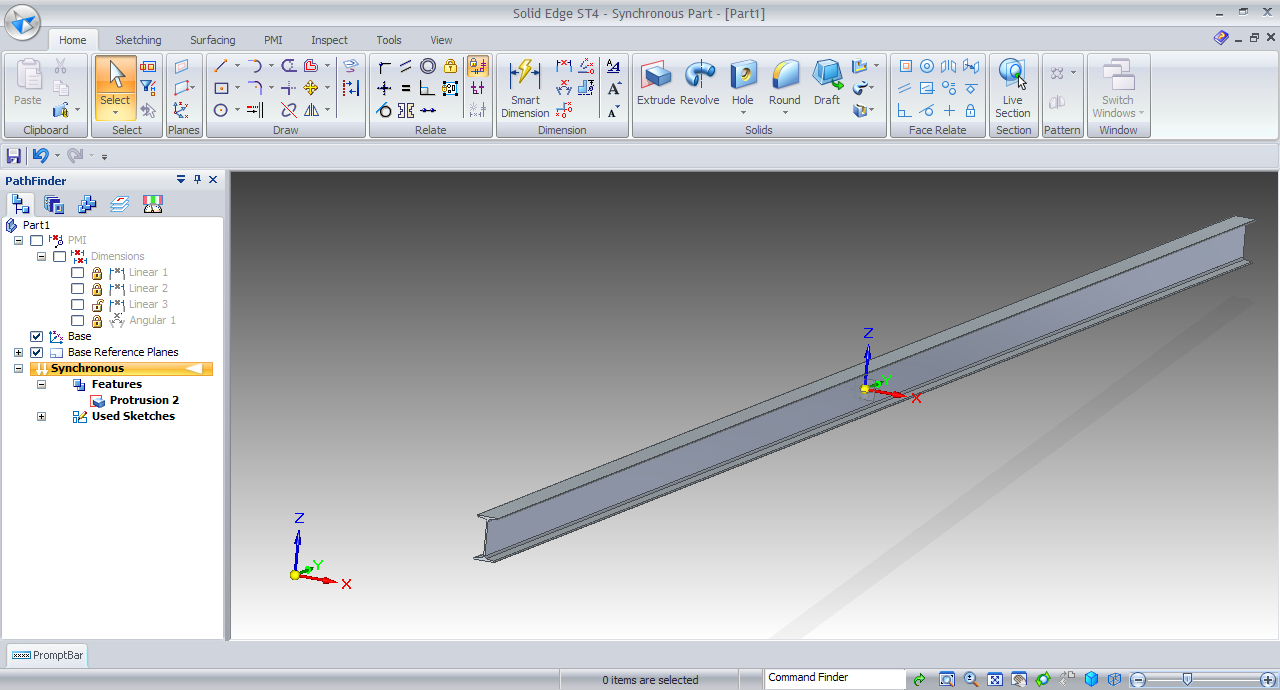# Tutorial on Modeling Structural beam 240x36 in Solid Edge?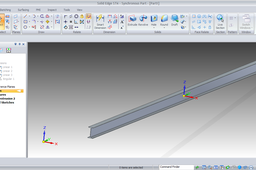Here is the tutorial on Modeling Structural Beam 240x36 ISO. In this tutorial we will learn how to make an ISO Structural Beam 240x36 in Solid Edge Synchronous Mode.

1. ### Step 1:

Start Solid Edge in Part mode.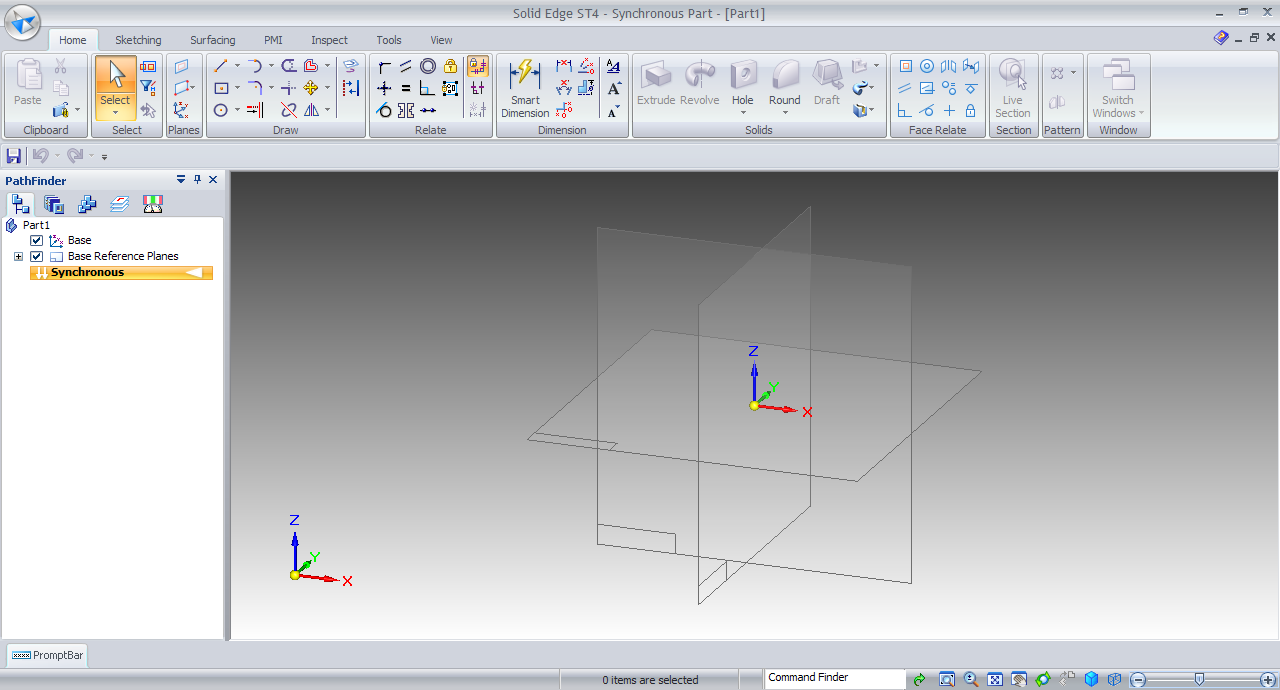2. ### Step 2:

CTRL + F for front view.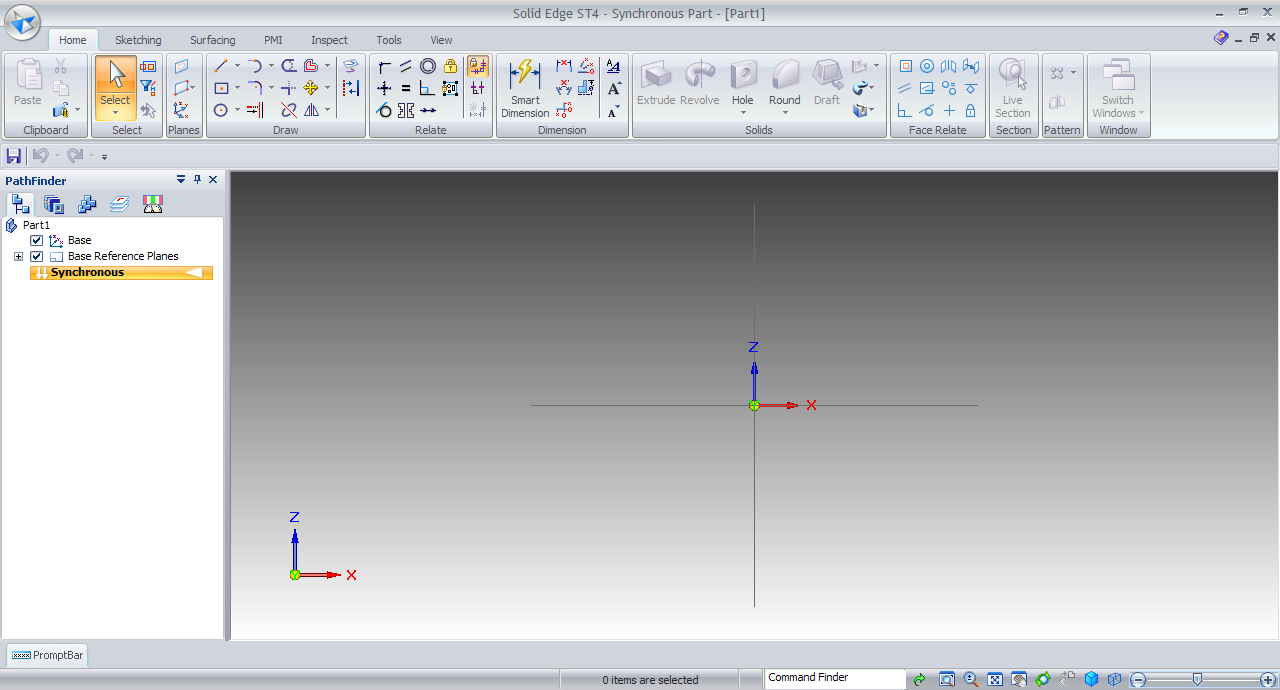3. ### Step 3:

Choose Line tool.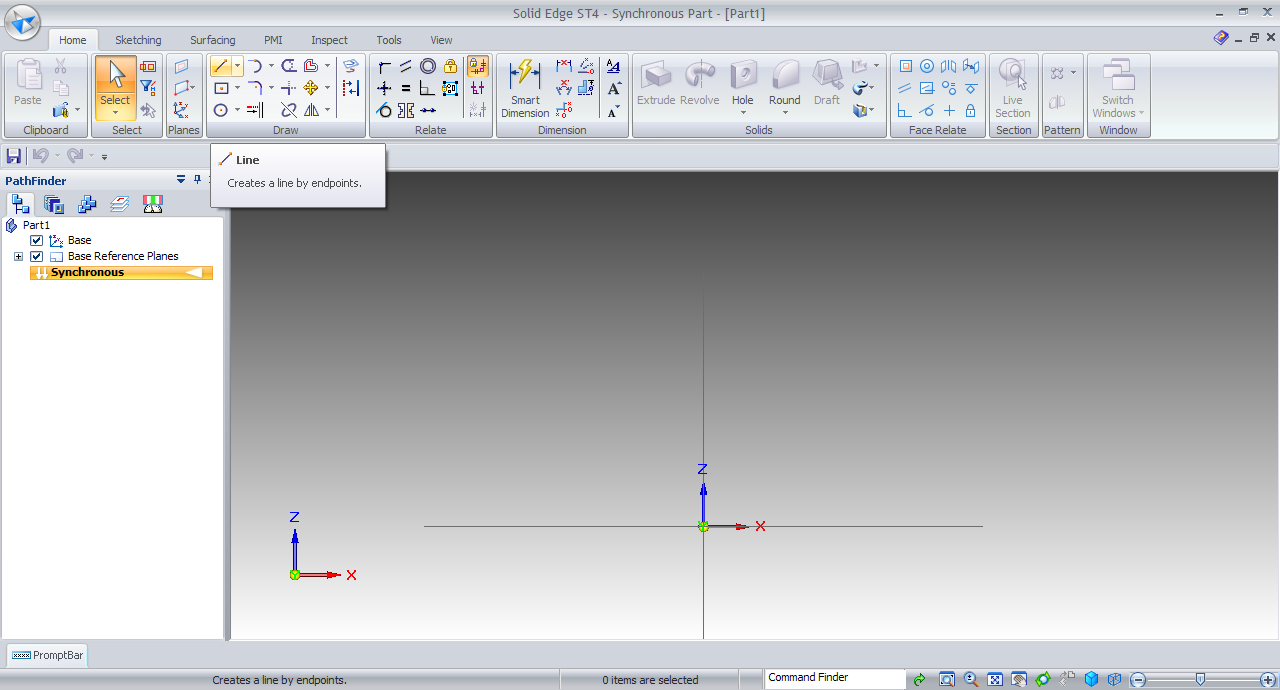4. ### Step 4:

Click for first end point along X-axis.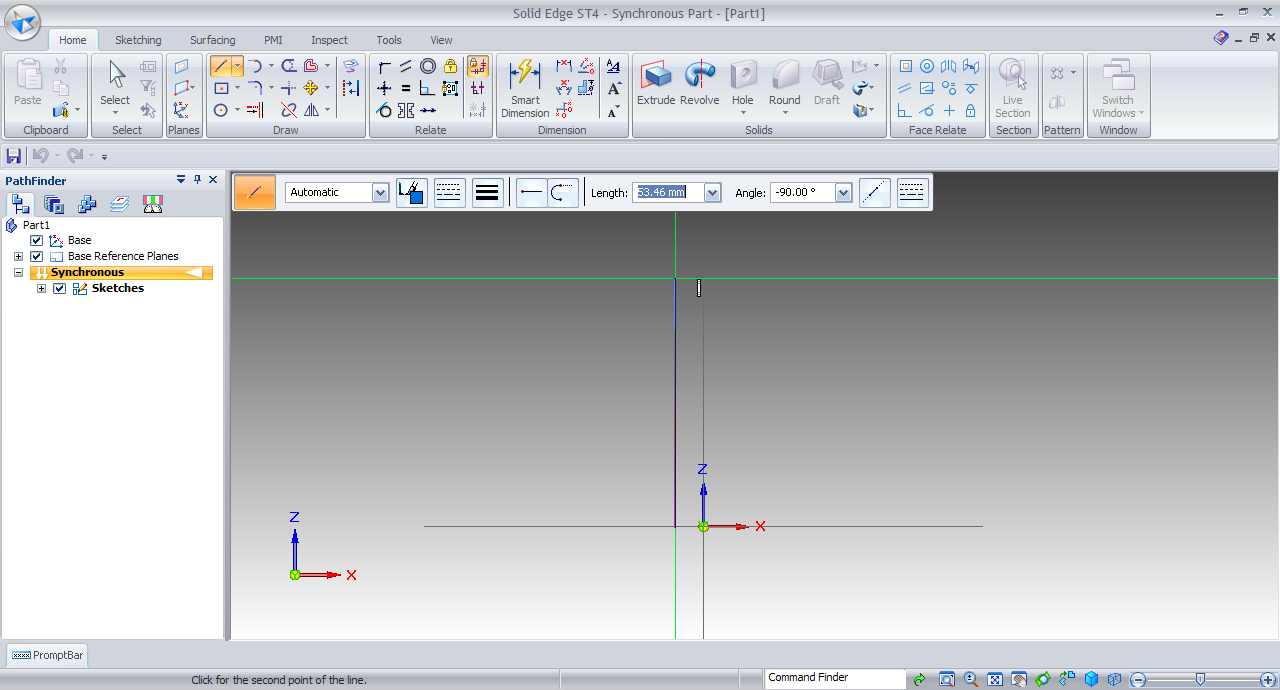5. ### Step 5:

Given length is 93.16mm.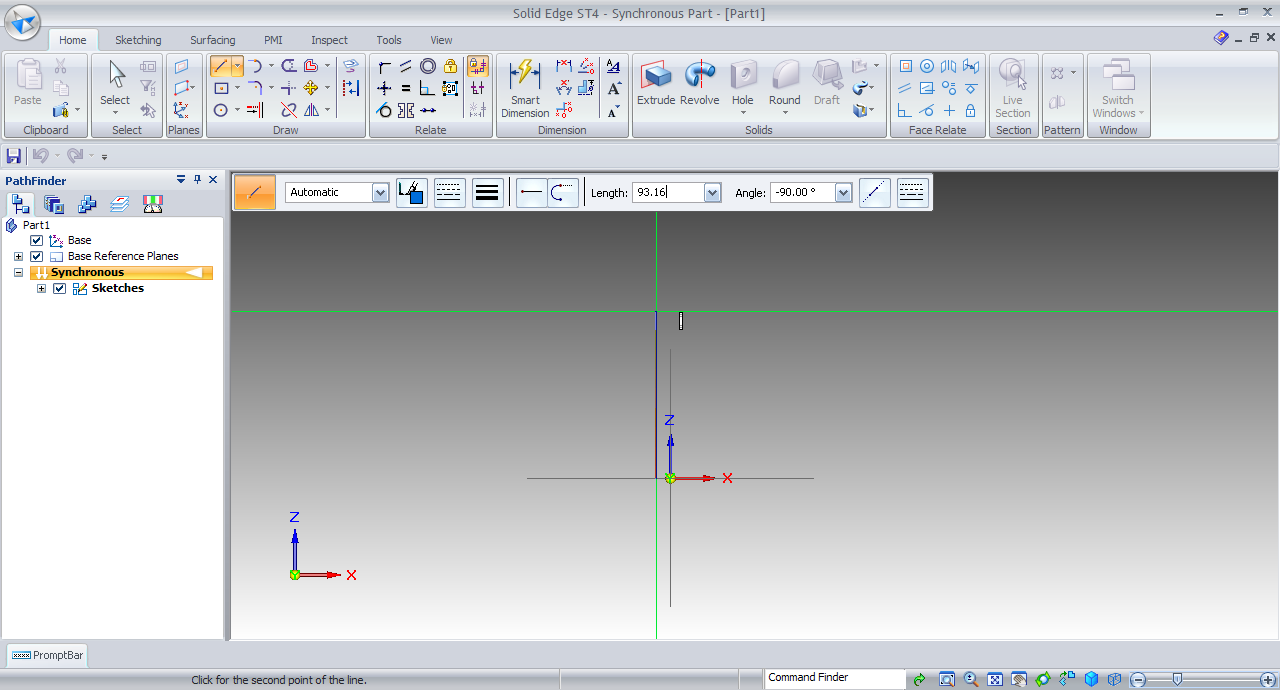6. ### Step 6:

Smart dimension this line with Z-axis at 6.15mm.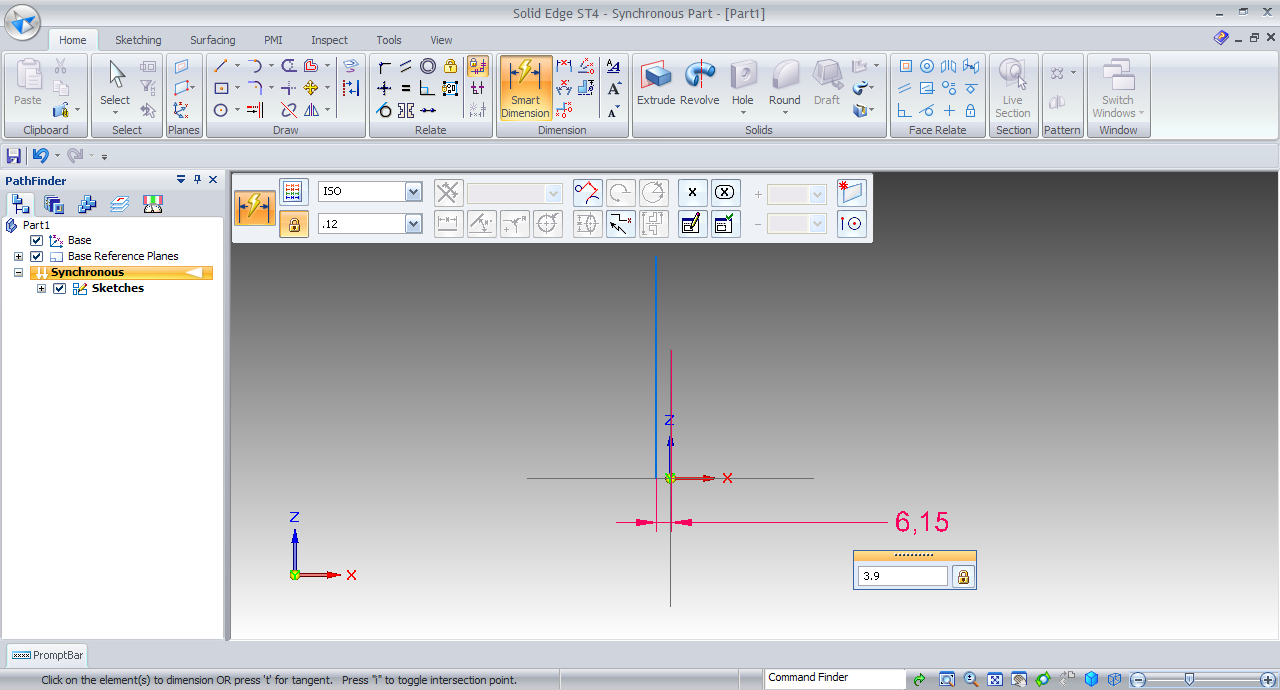7. ### Step 7:

Again Line and make a line end point along Z-axis.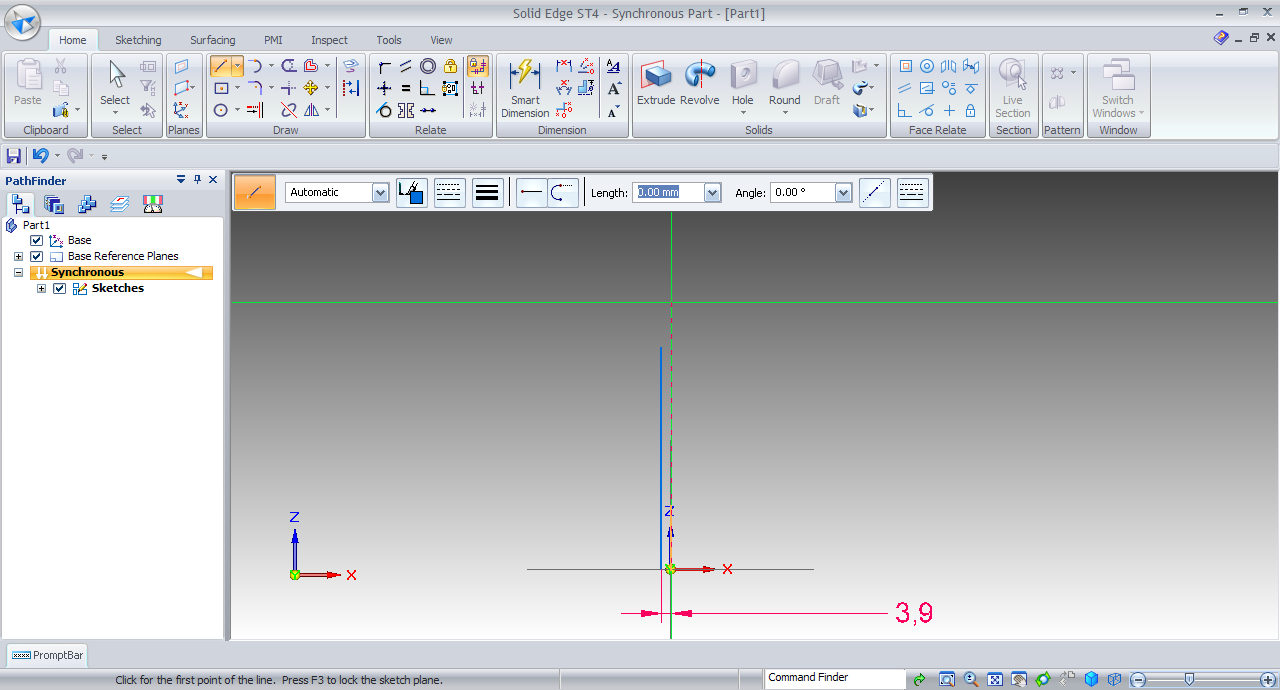8. ### Step 8:

Length be 60mm.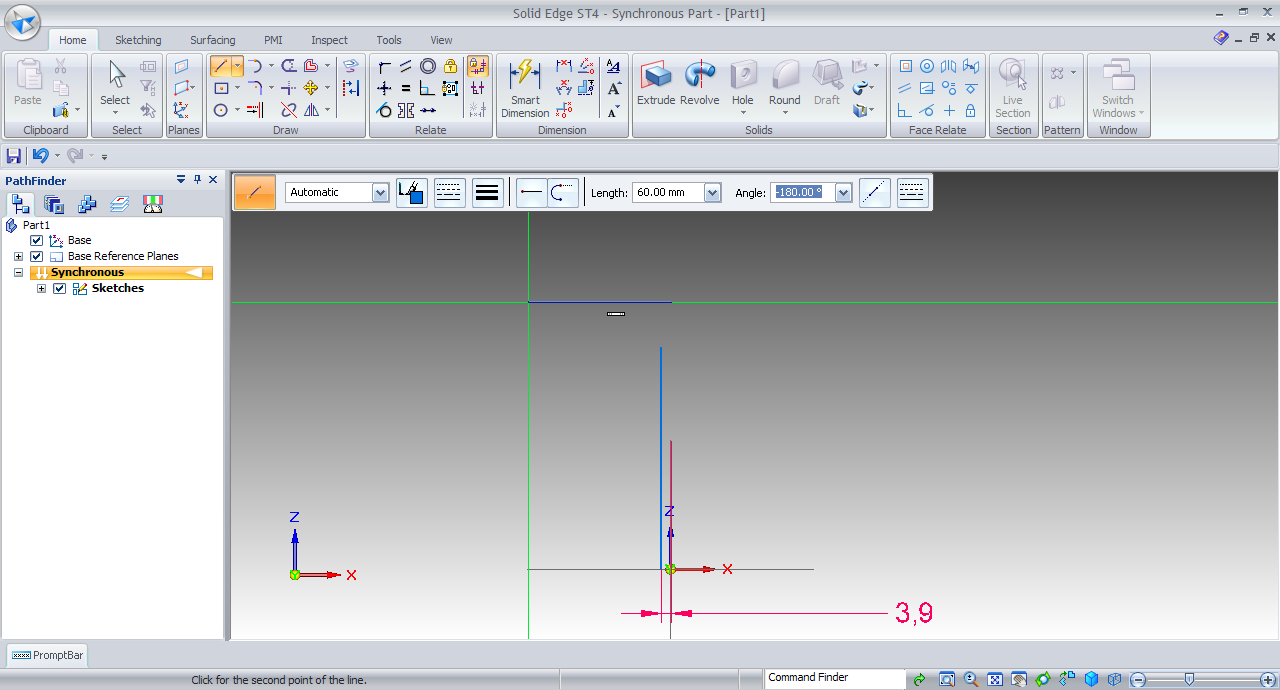9. ### Step 9:

Smart dimension this line with X-axis at 120mm.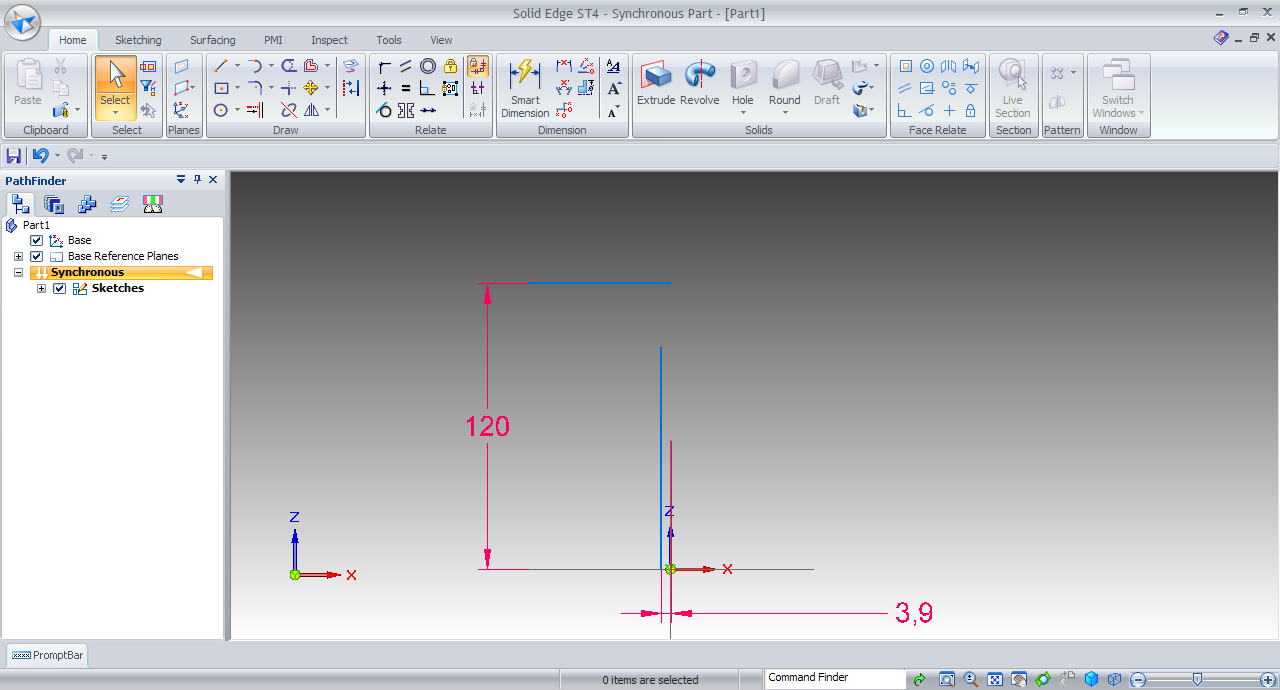10. ### Step 10:

Line tool and make the end point coincident with last line.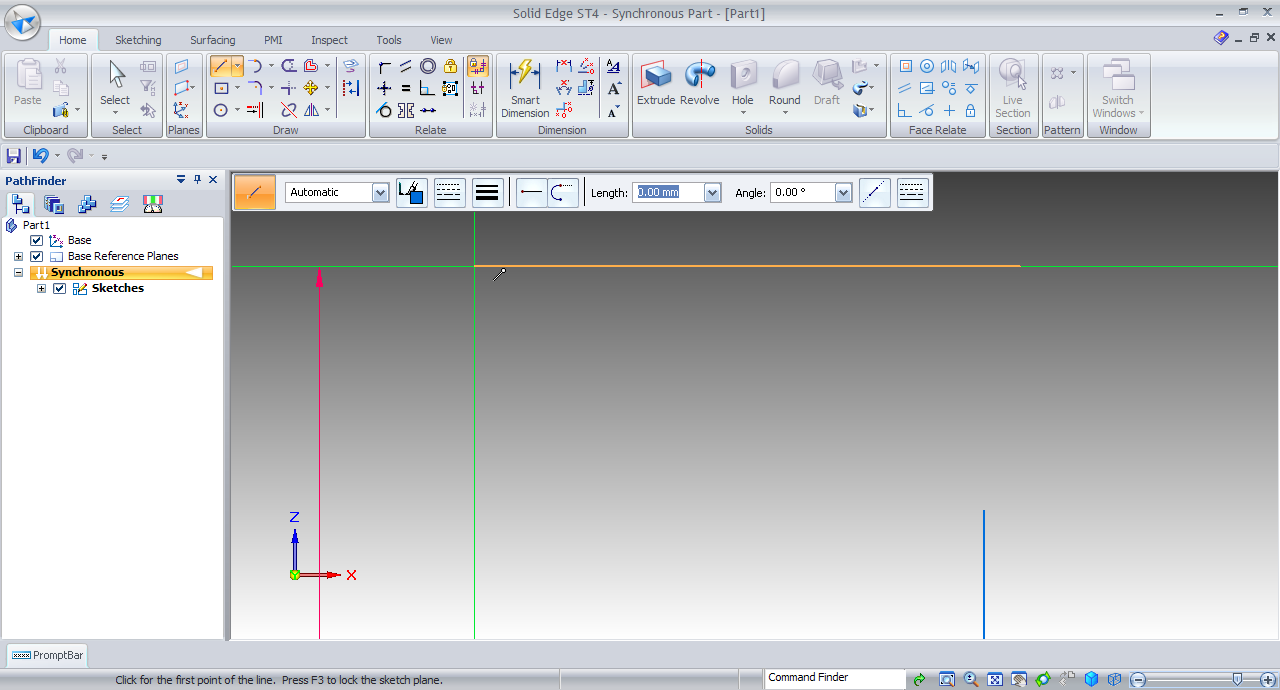11. ### Step 11:

Make line like this.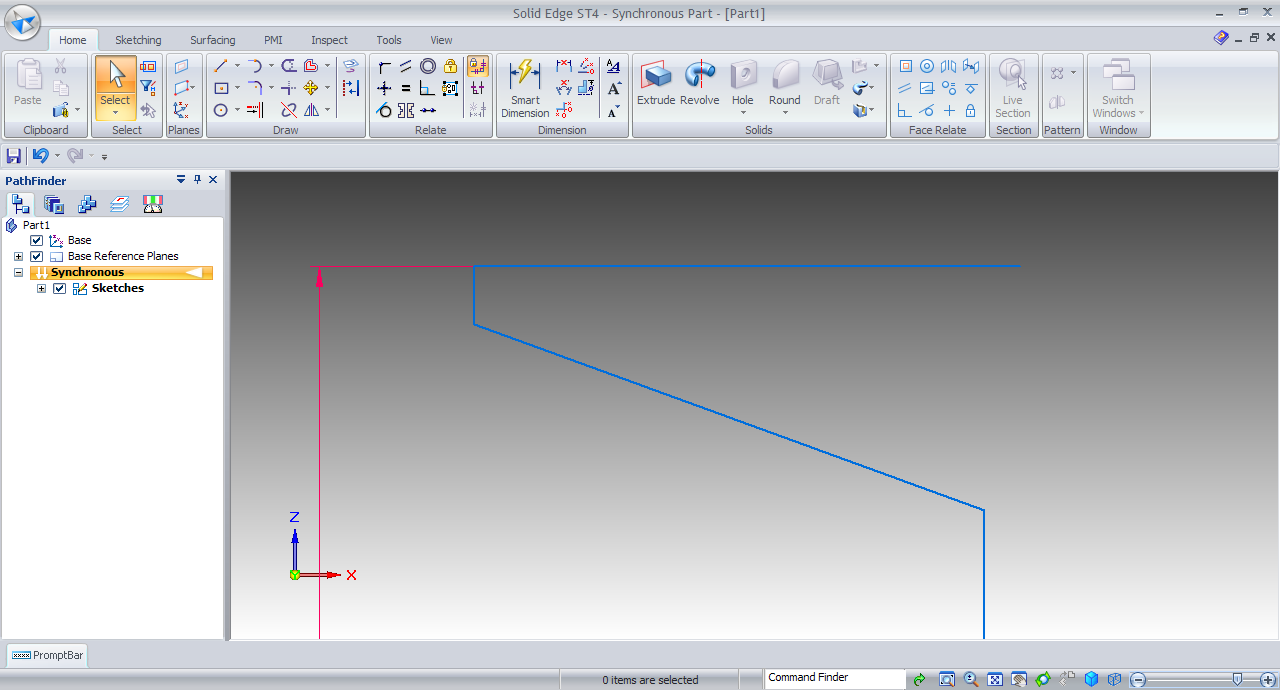12. ### Step 12:

Make angle b/w vertical and inclined line 99.46 degree.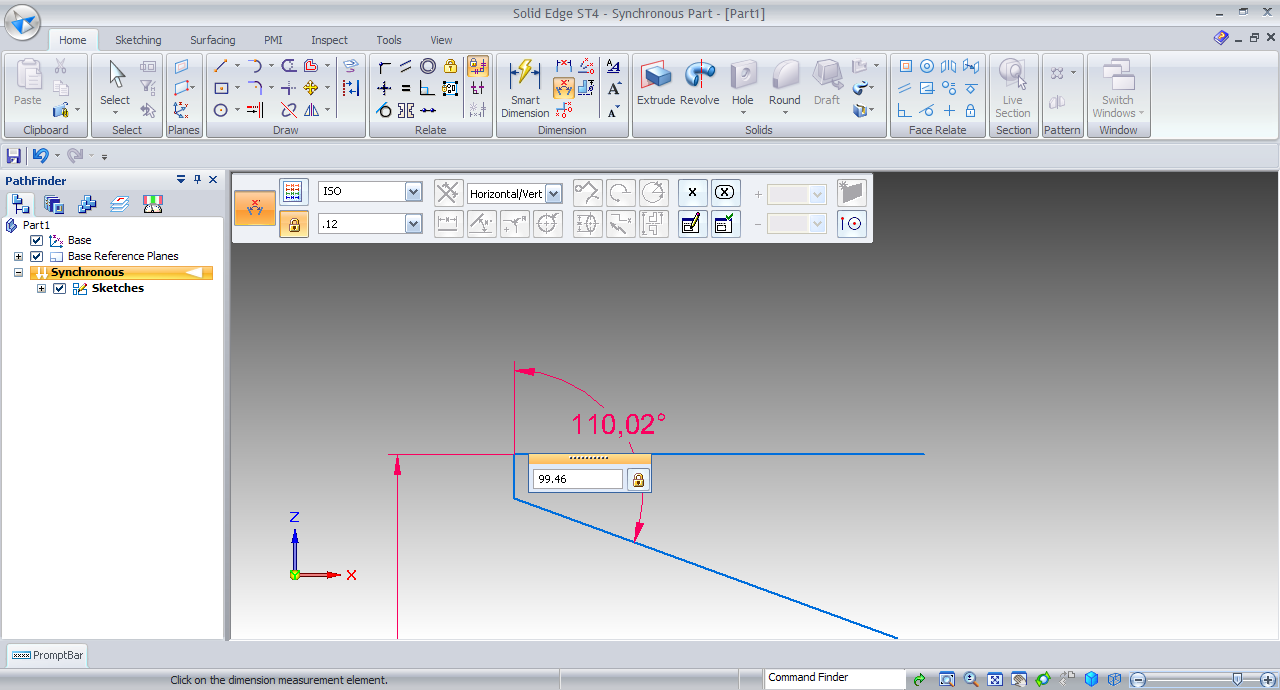13. ### Step 13:

Fillet the vertical and inclined line by 6mm.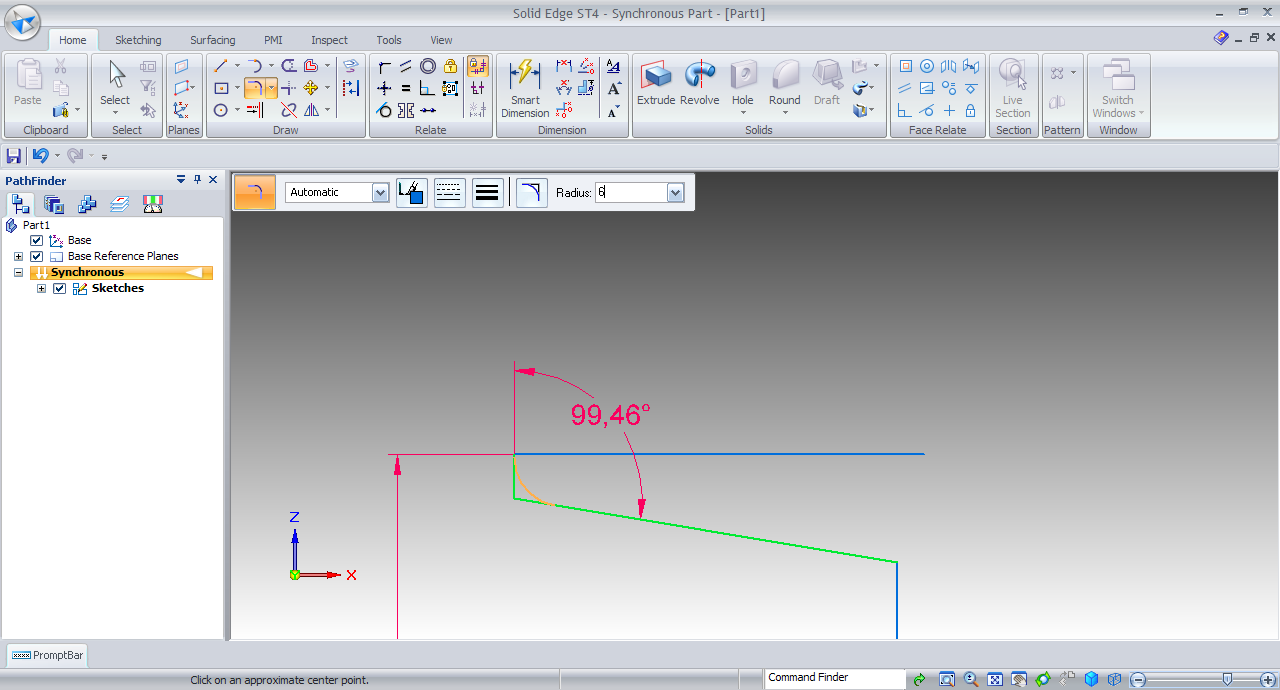14. ### Step 14:

Fillet the other line by 12mm.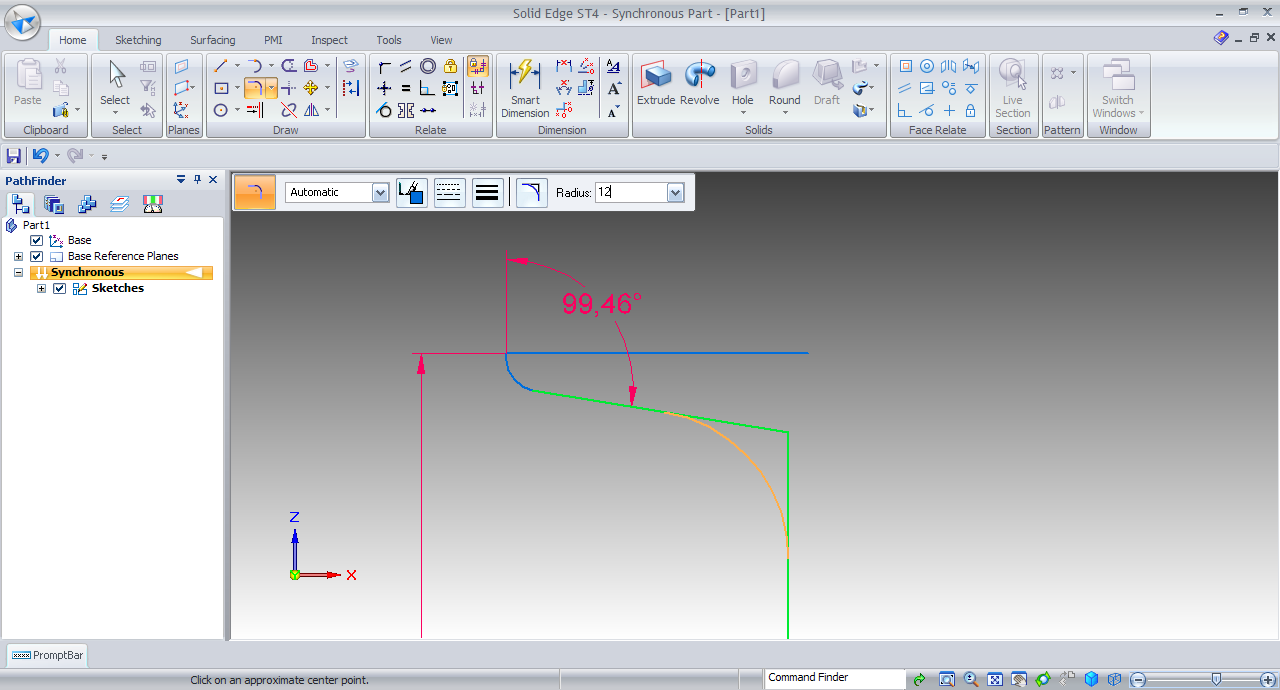15. ### Step 15:

Select Mirror.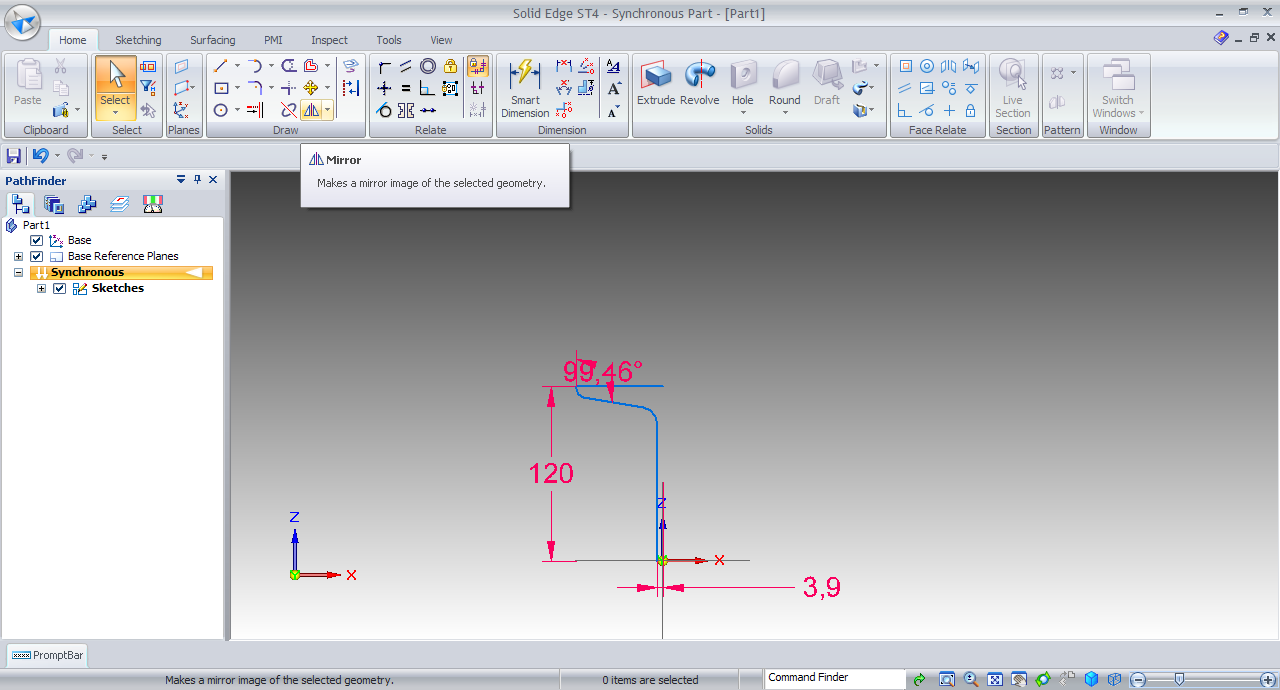16. ### Step 16:

Select all entities.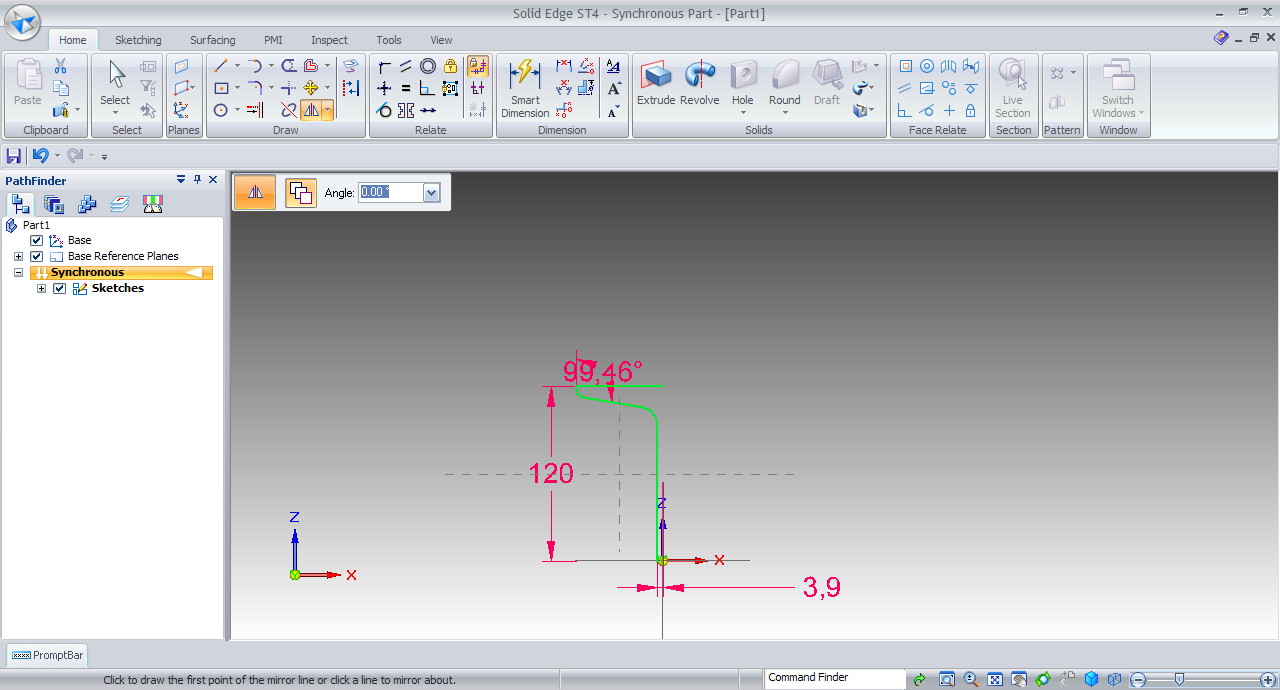17. ### Step 17:

Right click and then select Z-axis.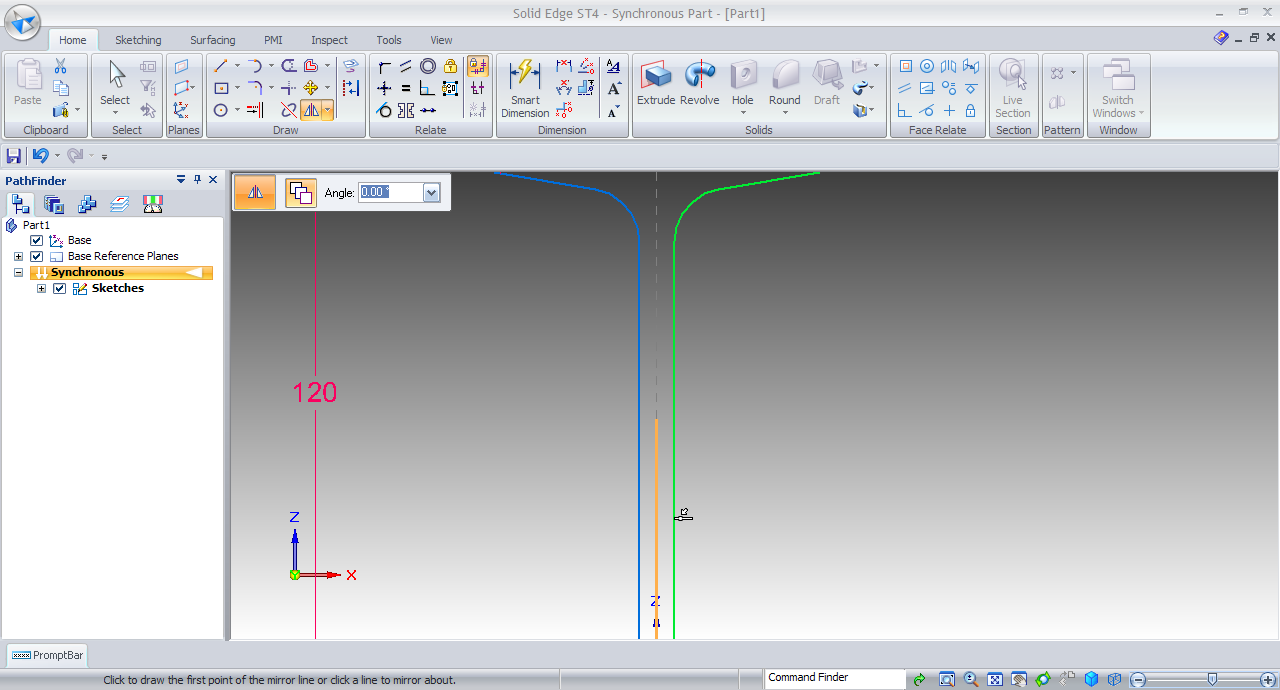18. ### Step 18:

Again mirror all entities about X-axis.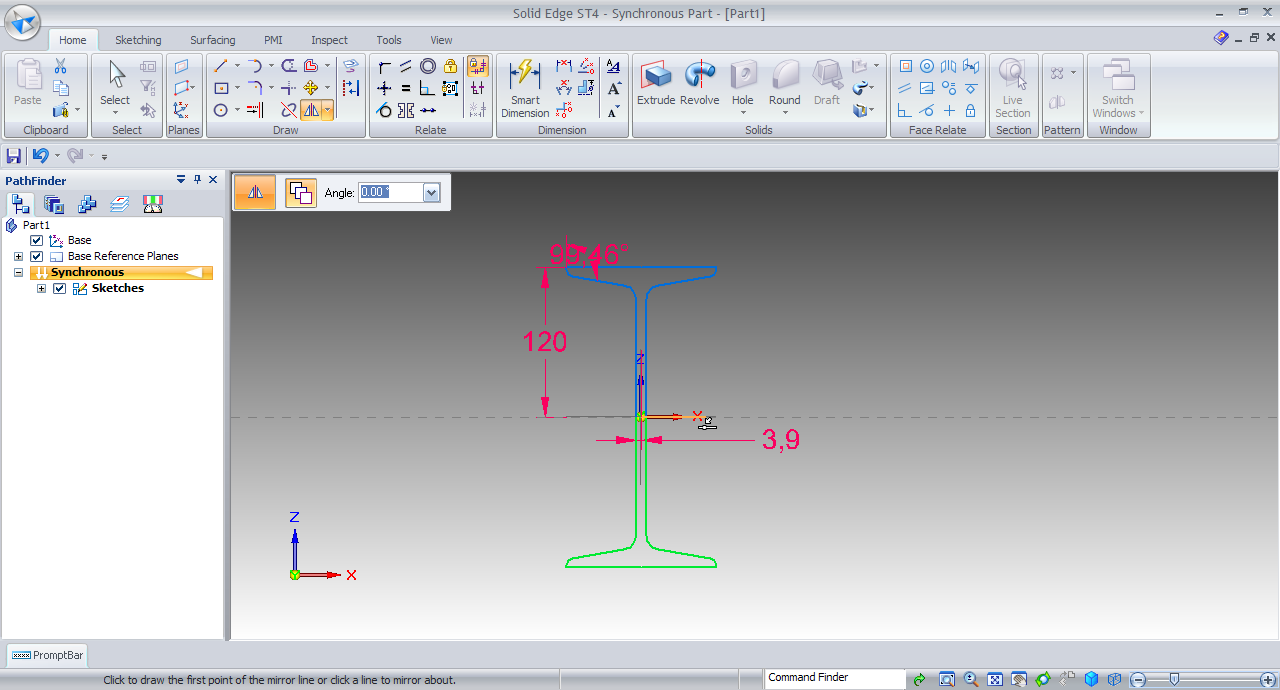19. ### Step 19:

CTRL+I for SE Isometric View.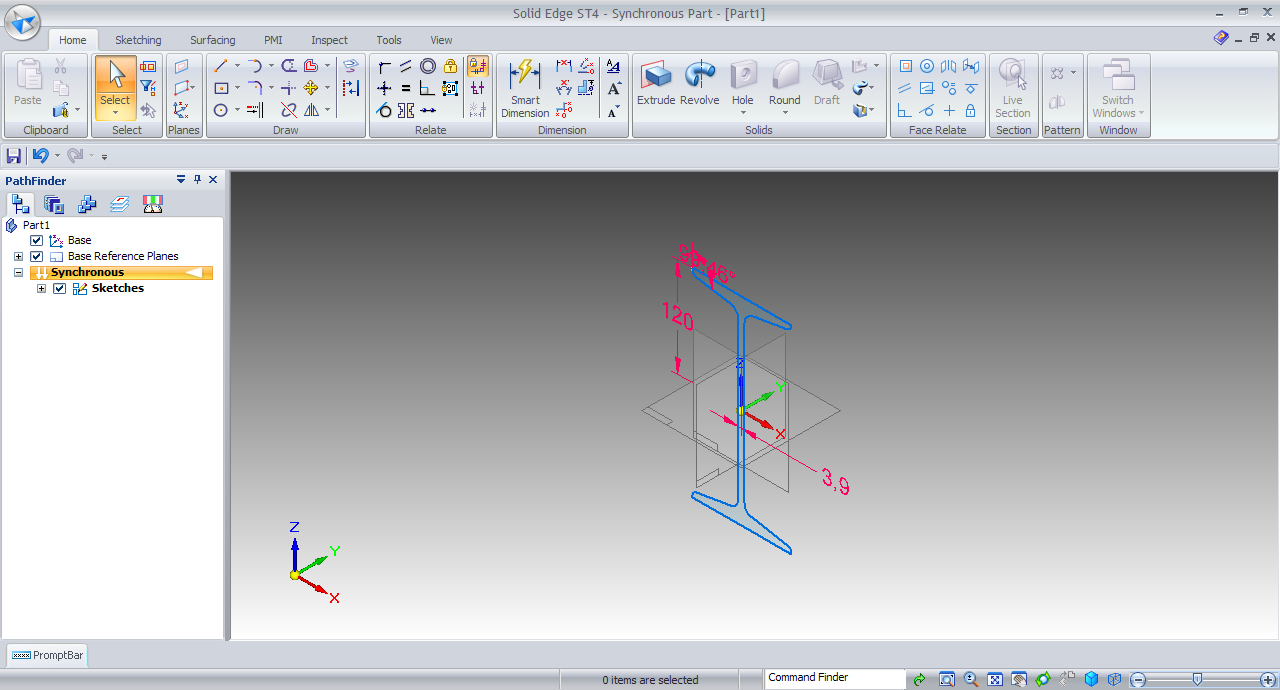20. ### Step 20:

Extrude tool.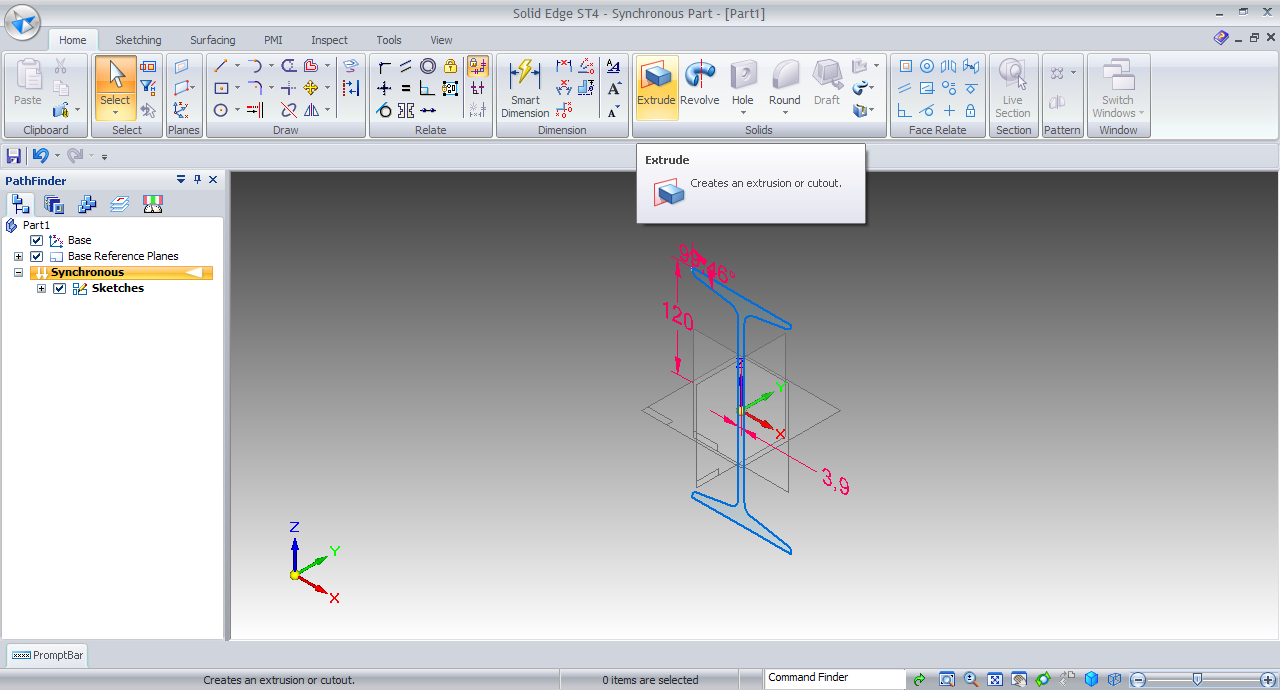21. ### Step 21:

Select the inside region of the Sketch.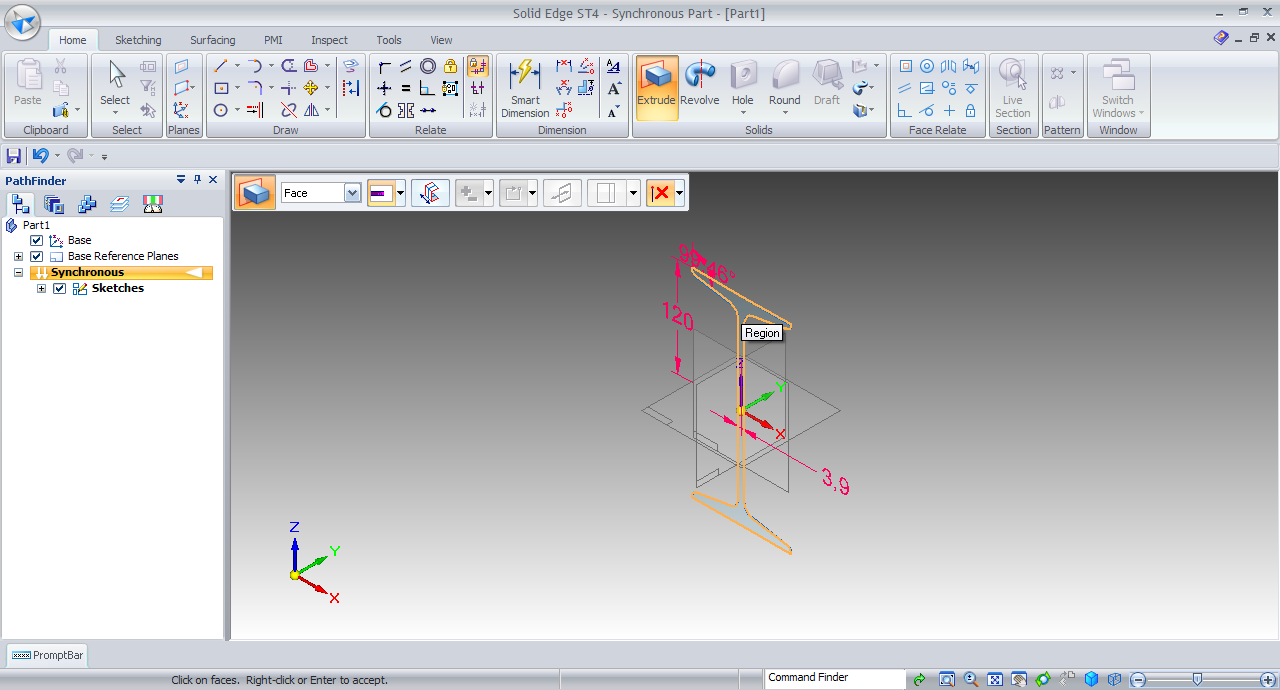22. ### Step 22:

Press shift for toggling Symmetric and input length be 10000mm i.e. 1m.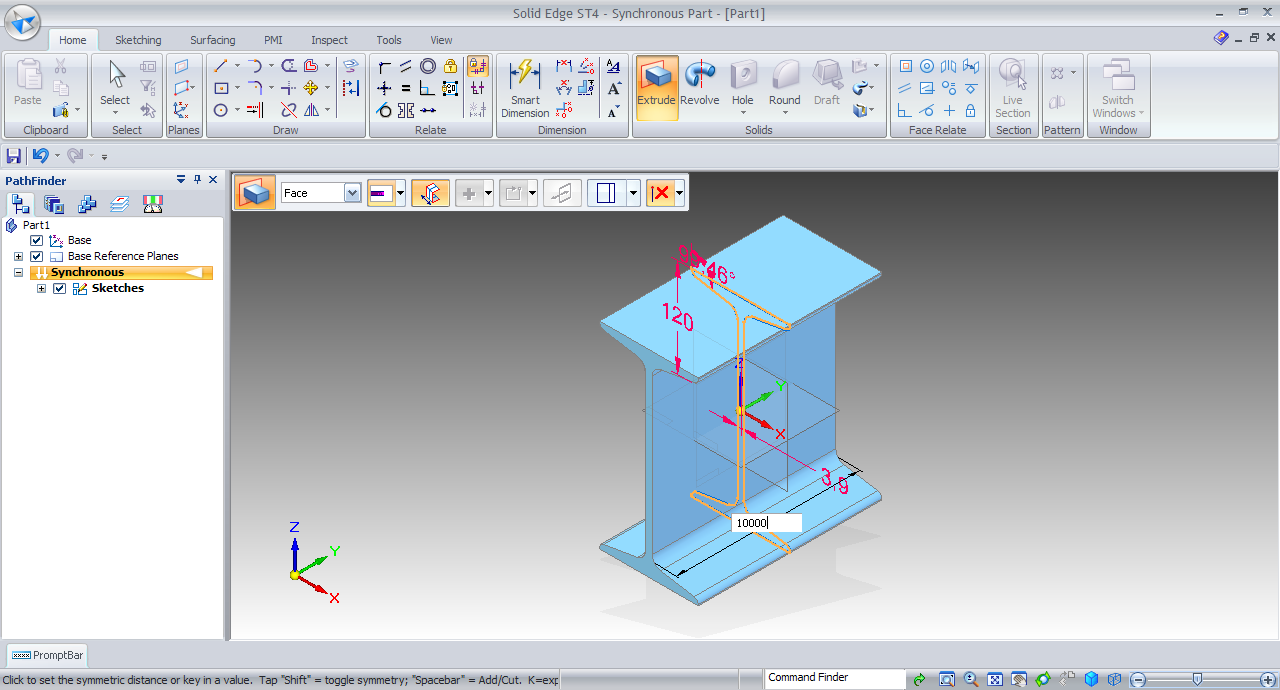23. ### Step 23:

And the body is generated.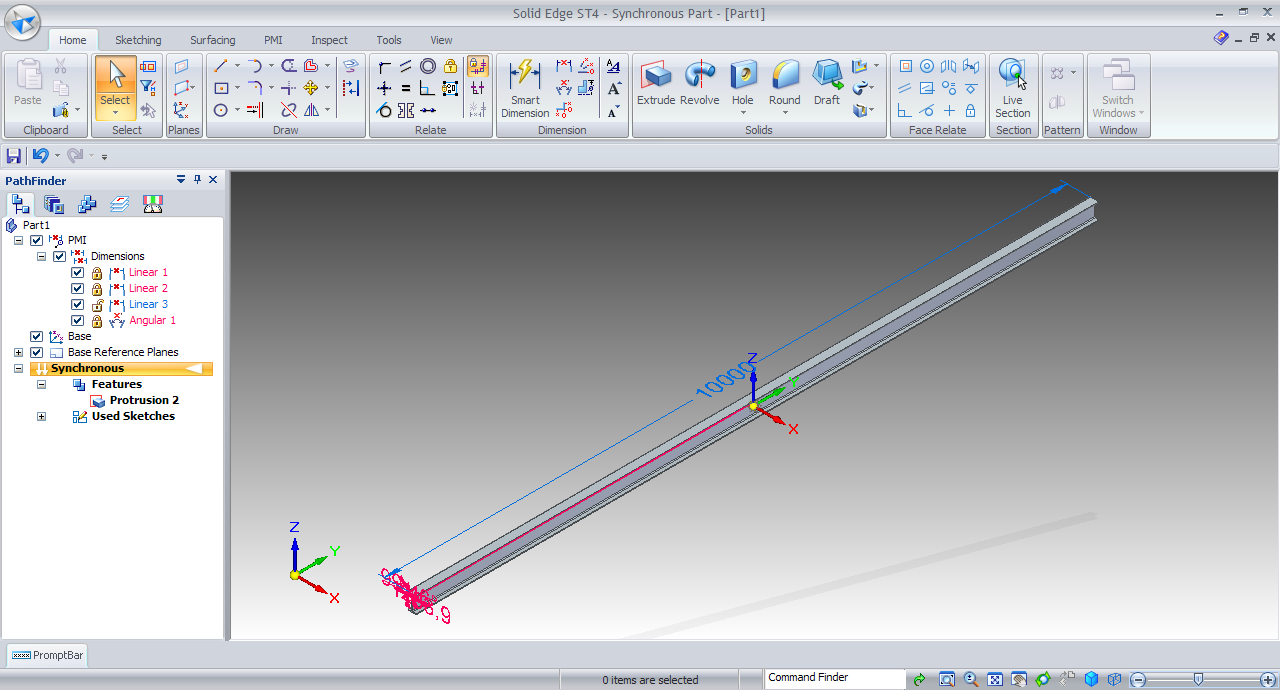24. ### Step 24:

Hide dimensions from path finder and the structural beam is ready.